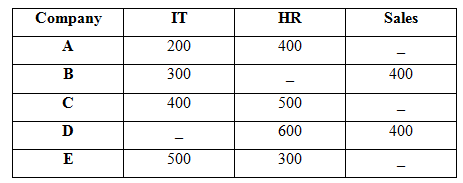# SBI Clerk Prelims 2021 Quantitative Aptitude Questions (Day-08)

Dear Aspirants, Our IBPS Guide team is providing new series of Quantitative Aptitude Questions for SBI Clerk Prelims 2020 so the aspirants can practice it on a daily basis. These questions are framed by our skilled experts after understanding your needs thoroughly. Aspirants can practice these new series questions daily to familiarize with the exact exam pattern and make your preparation effective.

Start Quiz

Table chart

Directions (1-5): Study the following information carefully and answer the questions given below.

The given table shows the number of employees of three different departments in 5 different companies.1) If total number of employees in C is 1200, then what is the difference between the total number of Sales employees from C and D?

A.100

B.150

C.200

D.250

E.None of these

2) If the number of Sales employees from A, C and E in the ratio of 3: 3: 4 and the total number of sales employees from all the companies together is 1800, then what is the total number of employees from E?

A.1000

B.1200

C.1500

D.1400

E.None of these

3) If the number of sales employees from A and C is equal and the total number of employees from C is 1200, then what is the total number of employees from A?

A.600

B.800

C.900

D.1000

E.None of these

4) If the total number of IT employees from all the companies together is 1900, then the number of IT employees from D is what percent more than the number of IT employees from C?

A.20%

B.25%

C.15%

D.30%

E.None of these

5) If the total number of HR employees from all the companies together is 1900, then what is the total number of employees from B?

A.800

B.700

C.600

D.900

E.None of these

Profit and loss

6) A seller gives 15% discount on an item, if he marked up price of item 40% above C.P and while selling he cheats the buyer by giving 10% less in weight. Find this overall profit percent (approximately).

A.32%

B.69%

C.45%

D.89%

E.97%

Boat and stream

7) Downstream speed of a boat is 8/5 times the upstream speed of the boat. The speed of the current is what percent of the speed of the boat in still water?

A.23%

B.36%

C.57%

D.78%

E.39%

Probability

8) A bag contains 3 white and 4 black balls. A second bag contains 3 white and 2 black balls. One bag is selected at random and from the selected bag one ball is drawn. Find the probability that the ball drawn is white.

A.6/7

B.12/13

C.18/35

D.5/6

E.None of these

Average

9) An average mark obtained by 100 students in an examination is 23. If the average mark of passed students is 35 and that of the failed students is 20, what is the number of students who passed the examination?

A.10

B.20

C.15

D.25

E.None of these

Ages

10) Ratio of present age of A and B is 5: 6. Sum of the present age of C and A is 41. D is 10 years younger than B. Average of present age of B, C and D is 22 years. Find the difference between D and C’s age.

A.2

B.4

C.1

D.3

E.None of these

Number of sales employees from C = 1200 – 400 – 500 = 300

Required difference = 400 – 300 = 100

3x + 3x + 4x + 400 + 400 = 1800

10x = 1000

x = 100

Number of sales employees in E = 4 * 100 = 400

Total number of employees in E = 400 + 500 + 300 = 1200

Number of sales employees in C = 1200 – 500 – 400 = 300 = Number of sales employees in A

Total number of employees from A = 300 + 400 + 200 = 900

Number of IT employees from D = 1900 – 200 – 300 – 400 – 500 = 500

Required percentage = (500 – 400)/400 * 100 = 25%

Number of HR employees from B = 1900 – (400 + 500 + 600 + 300) = 100

Total number of employees from B = 100 + 400 + 300 = 800

Let the CP be Rs.100.

MP = Rs.140 and SP = 85/100 * 140 = Rs.119

He cheats by giving 10% less in weight.

CP = 100 * 90/100 = Rs.90

Profit % = (119 – 90)/90 * 100 = 32.22% ≈ 32%

Let us take the speed of boat and speed of current be x and y respectively.

x + y = 8/5 * (x – y)

= > x/y = 13/3

Required percentage = 3/13 * 100 = 23%

Probability of selecting a bag = ½

Probability of getting white ball from first bag = 3/7

Probability of getting white ball from second bag = 3/5

Required probability = ½ * 3/7 + ½ * 3/5 = 3/14 + 3/10 = 18/35

Number of students passed be x.

Total marks = 100 * 23 = 35x + (100 – x) * 20

=> 2300 = 35x + 2000 – 20x

=> x = 20

Let the present age of A and B be 5x and 6x.

D = 6x – 10 and C = 41 – 5x

(B + C + D)/3 = 22

=> 6x + 41 – 5x + 6x – 10 = 66

=> x = 5

D’s age = 6x – 10 = 30 – 10 = 20

C’s age = 41 – 5x = 41 – 25 = 16

Required difference = D – C

= 20 – 16 = 4

 Check Here to View SBI Clerk Prelims 2021 Quantitative Aptitude Questions Day – 07 Day – 06 Day – 05